### 6.2.3 Ionization structure

Results for the fractional ionic abundances in the ring (torus) and inner (oblate spheroid) regions of our two models are shown in Table 9 and Fig. 11. It is clear from the figure and table that the ionization structures from the models vary through the nebula due to the complex density and radiation field distribution in the gas. As shown in Table 9 , He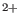/He is much higher in the inner regions, while He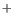/He is larger in the outer regions, as expected. Similarly, we find that the higher ionization stages of each element are larger in the inner regions. From Table 9 we see that hydrogen and helium are both fully ionized and neutrals are less than 8% by number in these best-fitting models. Therefore, our assumption of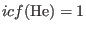is correct in our empirical method.

Table 10 lists the nebular average ionic abundance ratios calculated from the photoionization models. The values that our models predict for the helium ionic ratio are fairly comparable with those from the empirical methods given in §5, though there are a number of significant differences in other ions. The O/Hionic abundance ratio is about 33 per cent lower, while O/His about 60% lower in Model 2 than the empirical observational value. The empirical value of Sdiffers by a factor of 8 compared to our result in Model 2, explained by the shock-excitation effects on theII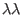6716,6731 doublet. Additionally, the Ne/Hionic abundance ratio was underestimated by roughly 67% in Model 2 compared to observed results, explained by the properties of the ionizing source. The Ar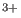/Hionic abundance ratio in Model 2 is 56% lower than the empirical results. Other ionic fractions do not show major discrepancies; differences remain below 35%. We note that the N/N ratio is roughly equal to the O/O ratio, similar to what is generally assumed in the(N) method. However, the Ne/Ne ratio is nearly a factor of 2 larger than the O/O ratio, in contrast to the general assumption for(Ne) (see equation 5). It has already been noted by Bohigas (2008) that an alternative ionization correction method is necessary for correcting the unseen ionization stages for the neon abundance.

Ashkbiz Danehkar
2018-03-26